# Texas Go Math Grade 7 Lesson 14.3 Answer Key Constructing a Net Worth

Refer to our Texas Go Math Grade 7 Answer Key Pdf to score good marks in the exams. Test yourself by practicing the problems from Texas Go Math Grade 7 Lesson 14.3 Answer Key Constructing a Net Worth.

## Texas Go Math Grade 7 Lesson 14.3 Answer Key Constructing a Net Worth

Identifying Financial Assets and Liabilities

Assets are the things you own. They have a positive cash value. Examples of assets are goods that are paid for and owned, such as houses, cars or bicycles, positive bank accounts, and savings bonds.

Liabilities are the debts you owe. They have a negative cash value. Examples of liabilities are car loans, house mortgages, credit card debt, or student loans.

For each description in the table below, decide whether the item is an asset or a liability. Explain your reasoning.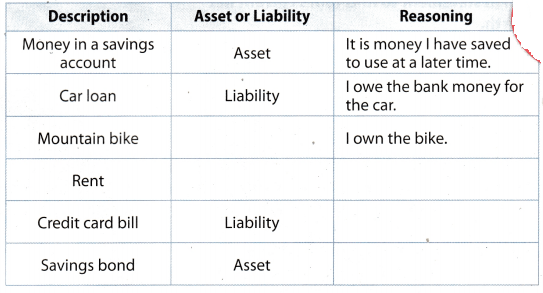Reflect

Question 1.
Critical Thinking How do you decide if something like a computer is an asset or a liability?
Computer is an asset
Because assets are those thing which we owe and it has positive cash value. So in case of computer once we buy it we own it And also computer has positive cash value. This means that computer is assets and when we will sell it we will get some cash.
Computer is an assets

Determine whether each described item is an asset or a liability. Explain your reasoning.

Question 2.
George leases a car for $175 per month. Answer: Leasing a car will be liability Because liabilities are those thing for which we have to pay and it has negative cash value. So in case of George who is leasing a car for$175 per month. lie has to pay $175 each month and this amount will be considered as the fixed exp expense. So every month owes the debit of$175 which means leasing a car is liabilities.
Leasing a car wilL be Liability

Question 3.
Morgana pays cash for a new flat screen television.
Paying cash for new teLevision is asset
Because assets are those thing which we owe and it has positive cash value. So in case of buying new television once we buy it we own it. And also the television has positive cash value. This means that television is assets and when we will sell it in future we will get some cash.
Paying cash for new television is asset

Example 1

Use the information below to construct a net worth statement to find Toni’s current net worth.

Toni paid off her motorcycle, which is worth $6,000. She owes 12 monthly installments of$65 each for her computer. She pays $45 per month for her cell phone and her contract lasts 1 year. She has$584 ¡n her checking account and $1,255 in her savings account. Toni owes$173 on her credit card. Her electronic appliances are valued at $1,641. Her share of the rent and utilities for the apartment she shares with roommates is$425 per month, and her lease lasts for another 6 months.

Step 1 List and total Toni’s assets.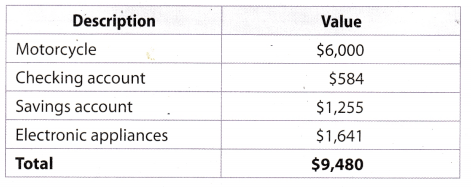Step 2 List and total Toni’s liabilities.Step 3 Subtract total liabilities from total assets to find Toni’s net worth.
Net worth = value of assets – value of liabilitiesToni’s current net worth is $5,437. Reflect Question 4. Critical Thinking Why is the monthly payment for Toni’s computer loan multiplied by 12 to find the total liability? Answer: Monthly installment of Toni’s computer loan =$65
Yearly instalment of computer 12 × $65 =$780
Here we muLtipLied the monthly installment of computer with 12 because when we are finding the total Liabilities or assets the calculations are done on yearly basis. In this case we are given with monthly payment of computer loan
so to find the total loan payment of computer in a year we have to multiply it by 12.
Because total liability are calculated on yearly basis.

Question 5.
What If? What if Toni did not own her motorcycle? Suppose she bought it with a loan and must make monthly payments of $240 per month for 2 more years. How will this change her current net worth? Explain. Answer: If did now own her motorcycle and she bought it with loan a loan of$240 per month then in his assets list $600 will be Subtracted and in liabilities list 12 × 24 =$2880 will he add. The calculation is shown below:
Current value of assets = $9,480 –$6000 = $3,480 Current value of lininlities =$4.043 + $2880 =$6,923
Current Net Worth = Value of assets – value of liabilities
= $3480 –$6923
= -$3443 Hence, current net worth of Toni is -$3443

Question 6.
Andre owns a condominium with a value of $145,000. He has a stock portfolio worth$8,300. He owes $3,700 on his car, which is valued at$9,500. He has $7,200 in student loans to repay. He has a credit card balance of$4,372. He also has $2,600 in a bank account. Construct a net worth statement to find Andre’s net worth. Answer: List of Andre total assets: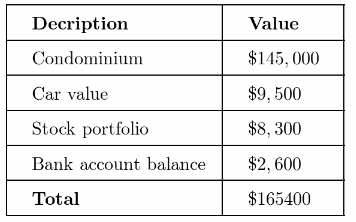List of Andre total liabilities: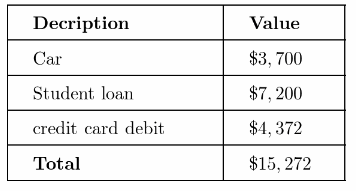Net worth = Value of assets – Value of liabilities =$165,400 $15,272 =$150, 128
Hence, Andre net worth is $150,128 Texas Go Math Grade 7 Lesson 14.3 Guided Practice Answer Key Question 1. Complete the table below. For each description, either decide whether the item is an asset or a liability or explain the reasoning. (Explore Activity)Answer: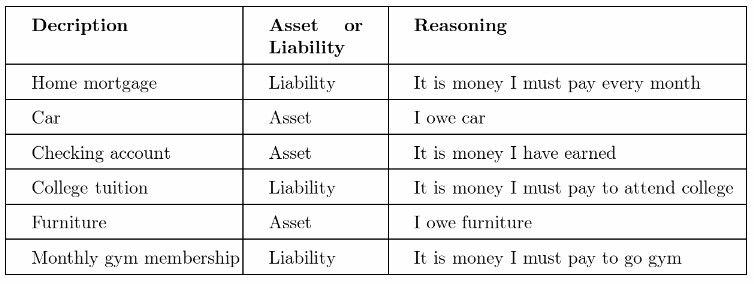Home mortgage is considered as liability Question 2. Rachel lives in a home with a value of$195,000 and has a mortgage of $160,000. She has electronic equipment worth$2,325. She is paying off the equipment with a loan of $875. Her car, which she owns, is worth$9,300. She has $6,890 in a bank account and$1,437 on her credit card. She owes $4,800 in student loans. She has a piano worth$1,200. Construct a net worth statement to find Rachel’s net worth. (Example 1)
Step 1 List Rachel’s assets and their values.
Step 2 Find the total of Rachel’s assets. ______
Step 3 List Rachel’s liabilities and their values.
Step 4 Find the total of Rachel’s liabilities.____
Step 5 Find Rachel’s net worth.
Net worth = ___________
Rachel’s net worth is ____.
List of Rachel’s total assets: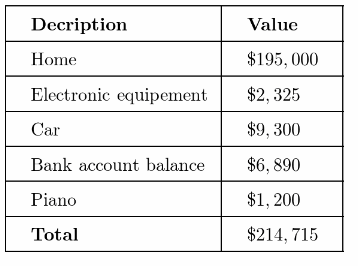List of Rachel’s total liabilities :Net worth = Value of assets – Value of liabilities
= $214, 715$167. 112
= $47,603 Hence, Rachel’s net worth is$47, 603

Essential Question Check-In

Question 3.
How can you calculate your net worth?
To calculate my net worth first i will list my all the assets and all the liabilities. Then we will calculate the current
values of my all the assets and liabilities. Once we are done with the current values of my all assets and liabilities,
we will add the total value of assets and total values of Liabilities separately. At last to find my net worth i will subtract the total value of Liability from the total assets value.
Net Worth = Value of assets – Value of Liabilities

Question 4.
Communicate Mathematical Ideas Travis pays cash for an e-reader. The value of the e-reader is $1,200. He buys a stereo system with his credit card. The value of the stereo system is$2,300. Is the e-reader an asset or a liability? Is the stereo system an asset or a liability? Explain.
e-reader will be asset for Tiavis because he has already paid the price of e-reader which is $1, 200. So now he owns the e-reader and it has some positive cash value which mean it will considered as asset. But in case of stereo system Travis has not paid the amount of stereo system by himself. Travis has paid through credit card so she owes the same amount which he has to pay in future. So currently stereo system lias negative cash value which means it will be consider as liability. e-reader is assets and stereo system is liability Question 5. Estimate your net worth. a. Create a table like the one below. List your assets. Include items such as your clothes, shoes, furniture, electronics (for example, a computer or a stereo), sports equipment, a musical instrument, money in a savings account, and savings bonds. Estimate the values.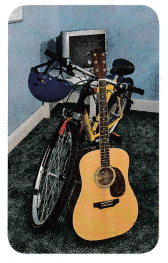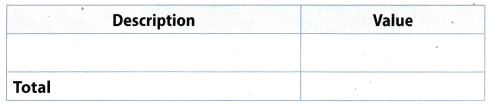Answer:b. Create a table like the one below. List your liabilities. Include items such as any money borrowed from your parents, pre-ordering video games, or belonging to a club with monthly dues. Estimate the values.Answer: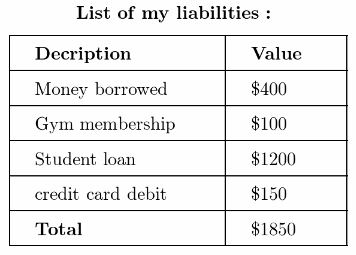c. What is your approximate net worth? ______ Answer: Net worth = Value of assets – Value of liabi[ities =$3,400 – $1,850 =$1,550
Hence, my net worth is $1550 My net worth is$1550

Question 6.
Analyze Relationships In 2011, according to the Federal Reserve, the median net worth of people underage 35 was $14,200. The median net worth of those from 35 to 44 was$69,400.
a. About how many times as great was the net worth of those from 35 to 44 as compared to those under 35?
Median net worth of people who under age 35 = $14, 200 Median net worth of people who who’s age 35 to 44 =$69, 400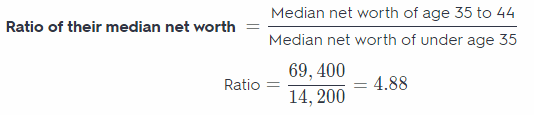Hence, the median net worth of people who’s age is between 35 to 4.88 times the median net worth of people who’s age is under 35.

b. Why do you think the median net worth is so much greater?
The median net worth is so much greater because after the age of 35 people start saving money and they are investing in deferent area such as owning the house or car etc. This way their net worth start increasing.
4.88 times

H.O.T. Focus on Higher Order Thinking

Question 7.
Critique Reasoning Paul has a stock portfolio worth $21,000. His home is valued at$325,000. His car is valued at $18,000. He owes$322,000 on his mortgage and $16,250 on his car loan. His credit card debt is$11,750. He owes student loans of $17,300. Because the value of his home and his car exceed their loans and his stock portfolio exceeds his credit card and student loan debt, Paul says he has a positive net worth. Is this true? Explain. Answer: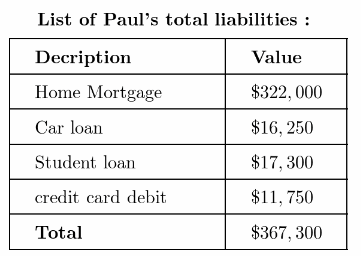Net worth = Value of assets – Value of Liabilities =$361, 000 $367, 300 = -$3,300
Hence, paul’s net worth is negative and it is – $3, 300 Question 8. Explain the Error Jessie wants to buy a guitar. She has enough money in her bank accounts to pay cash for a new guitar for$1,150 or a used guitar for $500. Jessie wants to choose the guitar that has a better impact on her net worth. Why is this not a good approach for making the decision? Answer: Choosing a guitar should not be based upon which one should have a better impact on the net worth This is because, for example, if you are to purchase a used guitar and yet the quality is not as good as a brand new guitar then over the time the used guitar couLd not be useful anymore and you wouLd purchase again a guitar Sometimes, purchasing an item should be based on its quality in order to save money because the Life span of the item will be longer. Purchasing an item should be based on its quality in order to save money because the life span of the item is longer. Question 9. Multistep Carla has a scooter worth$3,200. Her computer is worth $900. She owes$800 on her scooter and $483 on her credit card. Her personal property has a value of$2,100. She has $3,000 in her bank accounts and a mutual fund valued at$4,600. She wants to take out a $2,800 loan to buy some new appliances. The value of the appliances after purchase will go down 20%. How will the purchase of the appliances change Carla’s net worth? Explain. Answer: Determine the net worth of Carla. 3, 200 + 900 + (-800) + (-483) + 2, 100 + 3,000 + 4, 600 = 12, 517 The net worth of Carla is$12. 517. If she will take out $2,800 to purchase new appliances, her net worth will be: 12,517 – 2,800 = 9,717 If the purchased appliances will go down 20%, the appliances will be worth. Show 20% as decimal. 2. 800 × 0.20 = 560 2,800 – 560 = 2,240 Carla’s net, worth will then be: 9,717 + 2,240 = 11,957 The net worth of Carla will decreased by$560 once she purchased the new appliances because the value of the appliances will go down.
The net worth of Carla will decreased by \$560 because of the purchased new appliances.

Question 10.
Critical Thinking Do you think it is a good idea to track net worth monthly? Why?